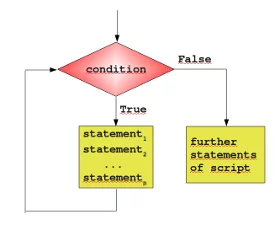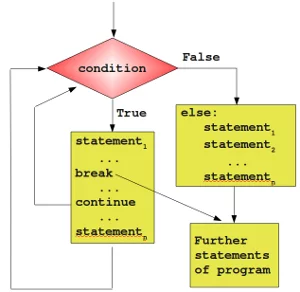## Loops### General Structure of a Loop

Many algorithms make it necessary for a programming language to have a construction which makes it possible to carry out a sequence of statements repeatedly. The code within the loop, i.e. the code carried out repeatedly, is called the body of the loop.

Essentially, there are three different kinds of loops:

• Count-controlled loops A construction for repeating a loop a certain number of times. An example of this kind of loop is the for-loop of the programming language C:

for (i=0; i <= n; i++)

Python doesn't have this kind of loop.

• Condition-controlled loop A loop will be repeated until a given condition changes, i.e. changes from True to False or from False to True, depending on the kind of loop. There are 'while loops' and 'do while' loops with this behaviour.

• Collection-controlled loop This is a special construct which allows looping through the elements of a 'collection', which can be an array, list or other ordered sequence. Like the for loop of the bash shell (e.g. for i in *, do echo \$i; done) or the foreach loop of Perl.Python supplies two different kinds of loops: the while loop and the for loop, which correspond to the condition-controlled loop and collection-controlled loop.

Most loops contain a counter or more generally, variables, which change their values in the course of calculation. These variables have to be initialized before the loop is started. The counter or other variables, which can be altered in the body of the loop, are contained in the condition. Before the body of the loop is executed, the condition is evaluated. If it evaluates to False, the while loop is finished. In other words, the program flow will continue with the first statement after the while statement, i.e. on the same indentation level as the while loop. If the condition is evaluated to True, the body, - the indented block below the line with "while" - gets executed. After the body is finished, the condition will be evaluated again. The body of the loop will be executed as long as the condition yields True.

### A Simple Example with a While Loop

It's best to illustrate the operating principle of a loop with a simple Python example. The following small script calculates the sum of the numbers from 1 to 100. We will later introduce a more elegant way to do it.

n = 100

total_sum = 0
counter = 1
while counter <= n:
total_sum += counter
counter += 1

print("Sum of 1 until " + str(n) +  " results in " + str(total_sum))

Sum of 1 until 100 results in 5050


### Exercise

Write a program, which asks for the initial balance K0 and for the interest rate. The program shall calculate the new capital K1 after one year including the interest.

Extend the program with a while-loop, so that the capital Kn after a period of n years can be calculated.

K = float(input("Starting capital? "))
p = float(input("Interest rate? "))
n = int(input("Number of years? "))

i = 0
while i < n:
K +=  K * p / 100.0
# or K *=  1 +  p / 100.0
i += 1
print(i, K)
print("Capital after " + str(n) + " ys: " + str(K))

1 100.6
2 101.2036
3 101.8108216
4 102.4216865296
5 103.0362166487776
6 103.65443394867026
7 104.27636055236228
8 104.90201871567645
9 105.53143082797051
10 106.16461941293834
Capital after 10 ys: 106.16461941293834


### The else PartSimilar to the if statement, the while loop of Python has also an optional else part. This is an unfamiliar construct for many programmers of traditional programming languages. The statements in the else part are executed, when the condition is not fulfilled anymore. Some might ask themselves now, where the possible benefit of this extra branch is. If the statements of the additional else part were placed right after the while loop without an else, they would have been executed anyway, wouldn't they? We need to understand a new language construction, i.e. the break statement, to obtain a benefit from the additional else branch of the while loop. The general syntax of a while loop looks like this:

while condition:
statement_1
...
statement_n
else:
statement_1
...
statement_n 
 while potatoes:
peel

cut
else:
pot pot on oven
cook for 40 minutes

and so on 

### Premature Termination of a while LoopSo far, a while loop only ends, if the condition in the loop head is fulfilled. With the help of a break statement a while loop can be left prematurely, i.e. as soon as the control flow of the program comes to a break inside of a while loop (or other loops) the loop will be immediately left. "break" shouldn't be confused with the continue statement. "continue" stops the current iteration of the loop and starts the next iteration by checking the condition. Here comes the crucial point: If a loop is left by break, the else part is not executed.

This behaviour will be illustrated in the following example, a little guessing number game. A human player has to guess a number between a range of 1 to n. The player inputs his guess. The program informs the player, if this number is larger, smaller or equal to the secret number, i.e. the number which the program has randomly created. If the player wants to gives up, he or she can input a 0 or a negative number. Hint: The program needs to create a random number. Therefore it's necessary to include the module "random".

import random
upper_bound = 20
lower_bound = 1
#to_be_guessed = int(n * random.random()) + 1
to_be_guessed = random.randint(lower_bound, upper_bound)
guess = 0
while guess != to_be_guessed:
guess = int(input("New number: "))
if guess > 0:
if guess > to_be_guessed:
print("Number too large")
elif guess < to_be_guessed:
print("Number too small")
else:
# 0 means giving up
print("Sorry that you're giving up!")
break   # break out of a loop, don't execute "else"
else:

Number too small

Number too large

Number too large

Number too large

Congratulations. You made it!


The previous program doesn't check if the number makes sense, i.e. if the number is between the boundaries upper_bound and over_bound. We can improve our program:

import random
upper_bound = 20
lower_bound = 1
to_be_guessed = random.randint(lower_bound, upper_bound)
guess = 0
while guess != to_be_guessed:
guess = int(input("New number: "))
if guess == 0:   # giving up
print("Sorry that you're giving up!")
break   # break out of a loop, don't execute "else"
if guess < lower_bound or guess > upper_bound:
print("guess not within boundaries!")
elif guess > to_be_guessed:
print("Number too large")
elif guess < to_be_guessed:
print("Number too small")
else:

Congratulations. You made it!


The boundaries should be adapted according to the user input:

import random
upper_bound = 20
lower_bound = 1
to_be_guessed = random.randint(lower_bound, upper_bound)
guess = 0
while guess != to_be_guessed:
guess = int(input("New number: "))
if guess == 0:   # giving up
print("Sorry that you're giving up!")
break   # break out of a loop, don't execute "else"
if guess < lower_bound or guess > upper_bound:
print("guess not within boundaries!")
elif guess > to_be_guessed:
upper_bound = guess - 1
print("Number too large")
elif guess < to_be_guessed:
lower_bound = guess + 1
print("Number too small")
else:

Number too small

Number too small

Number too large

Number too small

Congratulations. You made it!

import random
upper_bound = 20
lower_bound = 1
#to_be_guessed = int(n * random.random()) + 1
to_be_guessed = random.randint(lower_bound, upper_bound)
guess = 0
while guess != to_be_guessed:
guess = int(input("New number: "))
if guess == 0:   # giving up
print("Sorry that you're giving up!")
break   # break out of a loop, don't execute "else"
if guess < lower_bound or guess > upper_bound:
print("guess not within boundaries!")
elif guess > to_be_guessed:
print("Number too large")
elif guess < to_be_guessed:
print("Number too small")
else:

Number too small

Your guess is contradictory to your previous guesses!

Number too small

Your guess is contradictory to your previous guesses!

guess not within boundaries!

Number too small

Number too small

Number too small

Number too small

Congratulations. You made it!